## Introduction to Pandas Boxplots

A boxplot, or box-and-whisker plot, is a popular tool for visualizing the distribution of multiple sets of data at once. Like histograms and density plots, boxplots show the distribution of a given set of data. Unlike histograms and density plots, though, boxplots present a simplified illustration of the data. Boxplots are designed to help you quickly identify important quartile boundaries, which is valuable when you’re analyzing several datasets at once.

Pandas DataFrames come pre-equipped with methods of creating boxplots, making their preparation and presentation easy. We can create boxplots from Pandas DataFrames using the `pandas.DataFrame.boxplot` DataFrame method, which is a sub-method of `matplotlib.pyplot.boxplot`.

Pandas uses the Python module Matplotlib to create and render all plots, and each plotting method from `matplotlib.pyplot.boxplot` takes optional arguments that are passed to the Matplotlib functions. In this tutorial, we will cover the essential tools and options for plotting boxplots, but you should be aware that many more options (e.g. plot color, size, etc.) are available to be passed to the Matplotlib via `matplotlib.pyplot.boxplot`.

To illustrate creating boxplots, we will assume we have the following DataFrame `df` containing test grades already available within our Python environment:

``````df.info()
> <class 'pandas.core.frame.DataFrame'>
> RangeIndex: 746 entries, 0 to 745
> Data columns (total 3 columns):
> Test_1    746 non-null float64
> Test_2    746 non-null float64
> Type      746 non-null int64
> dtypes: float64(2), int64(1)
> memory usage: 17.6 KB

>        Test_1     Test_2  Type
> 0   85.868553  88.160763     2
> 1   88.017580  89.946783     3
> 2   76.811083  82.444485     3
> 3  100.000000  68.406203     3
> 4   94.719547  63.994755     2``````

Here we have two features, Test_1 and Test_2, along with a group number for each test given by the feature `Type`.

If we would like to create a fast set of boxplots for exploratory analysis, we can use the simple `pandas.DataFrame.boxplot` method:

``df.boxplot()``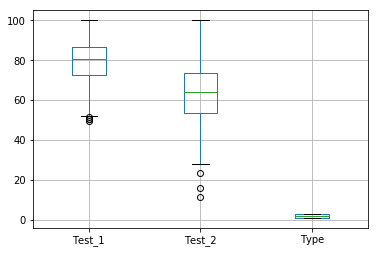This command produced boxplots for each of the 3 features we specified. Notice how it even creates a boxplot for our Type column, which we’re really not interested in analyzing! The Type is just supposed to assign each grade to group number.

So how do we make it so the Pandas boxplot doesn’t try to plot this extra column? To alter the default plots, we will have to specify additional options.

## Single Boxplot from a Pandas DataFrame

The simple `df.boxplot()` method above plotted boxplots of every feature in the DataFrame. If we wish to only examine a subset of the features, or even look at only one, then we should specify the `column` argument of the `df.boxplot()` method. The `column` argument takes either a string or list of strings of columns names:

``df.boxplot(column="Test_1")  # Plot a single column``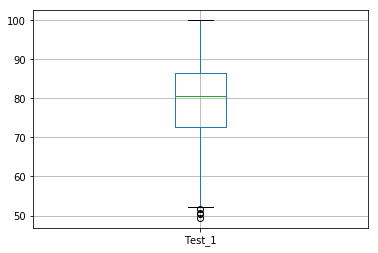Notice how the y-axis is autosized to fit the data from our Test_1 feature.

### Modifying Boxplot Orientation

Occasionally boxplots are plotted horizontally to show the spread of data over the x-axis. The orientation of the boxplots is given by the `vert` option, which accepts a boolean value to specify that the boxplots should be plotted with a vertical orientation. The default value is True. To plot horizontal boxplots, we simply set `vert=False`.

``df.boxplot(column="Test_1", vert=False)  # Plot a single column horizontally``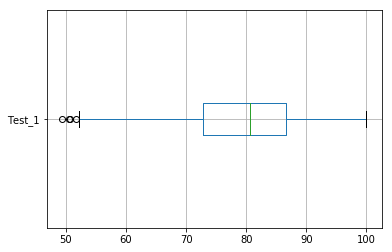I put together a Python Developer Kit with over 100 pre-built Python scripts covering data structures, Pandas, NumPy, Seaborn, machine learning, file processing, web scraping and a whole lot more - and I want you to have it for free. Enter your email address below and I'll send a copy your way.

Yes, I'll take a free Python Developer Kit

### Modifying Boxplot Width

Sometimes the box width of the output boxplot may need to be changed to fully express the given data. To manually change the width of the boxes, the `widths` option can be adjusted to a floating point width. For example, we can expand the width of our boxplot with the following:

``df.boxplot(column="Test_1", widths=0.5)  # Change width of the boxes``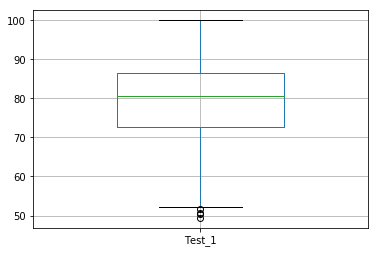### Modifying Boxplot Calculation Options

There are numerous ways to change how Matplotlib calculates and plots the boxplots, which are detailed in the manual page . However, it is highly recommended to use the default values, as box and whisker demarcations are standard across applications, and changing plotting parameters can grossly mislead your audience. Changes to these values should only be performed to bring your boxplots into a standard expected by your audience.

## Multiple Pandas Boxplots from a DataFrame

The `column` feature can also take a list of column names and produce separate plots for each chosen column. Take a look at this example:

``df.boxplot(column=["Test_1", "Test_2"])  # Plot specific columns``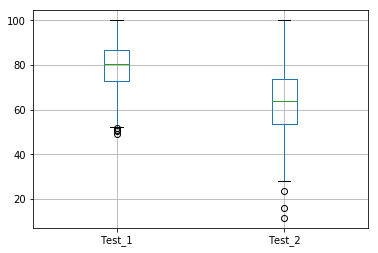In the example below, we told Pandas to create a boxplot for both Test_1 and Test_2 on the same figure.

### Grouping Pandas data with 'by' Option

Suppose our data is typed according to some common feature of the data, or that our data exists in categories. In these cases, we may want to determine the distributions of each type separately. Recall that in our example data the categories are given by the Type column. We can create multiple plots of data grouped by a common feature using the `by` option of the `pandas.DataFrame.boxplot` method.

The `by` option will take an object by which the data can be grouped. This can include a string of the column name specifying how you want to separate the data:

``df.boxplot(by="Type", column="Test_1")``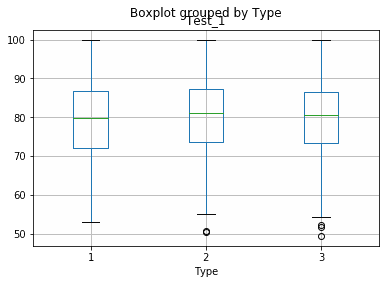We can also create multiple plots of different features based on a common `by` column by specifying additional features within the `column` option.

``df.boxplot(by="Type", column=["Test_1", "Test_2"])``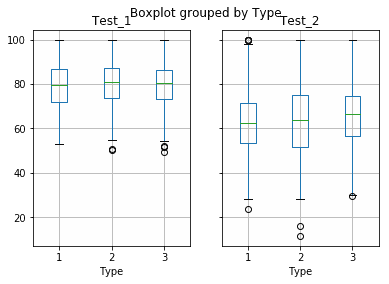Instead of plotting our Type column in our boxplot, like Pandas did in our default boxplot, we’ve now told Pandas to group our Test_1 and Test_2 data by this common column and show the box-and-whisker distribution. How cool is that? By digging into the optional Pandas boxplot arguments, you can create some neat visual representations of your data.